Courses

# MCQ (Practice) - States Of Matter (Level 2)

## 24 Questions MCQ Test | MCQ (Practice) - States Of Matter (Level 2)

Description
This mock test of MCQ (Practice) - States Of Matter (Level 2) for Class 11 helps you for every Class 11 entrance exam. This contains 24 Multiple Choice Questions for Class 11 MCQ (Practice) - States Of Matter (Level 2) (mcq) to study with solutions a complete question bank. The solved questions answers in this MCQ (Practice) - States Of Matter (Level 2) quiz give you a good mix of easy questions and tough questions. Class 11 students definitely take this MCQ (Practice) - States Of Matter (Level 2) exercise for a better result in the exam. You can find other MCQ (Practice) - States Of Matter (Level 2) extra questions, long questions & short questions for Class 11 on EduRev as well by searching above.
QUESTION: 1

### The ratio between the r. m. s. velocity of H2 at 50 K and that of O2 at 800 K is :

Solution: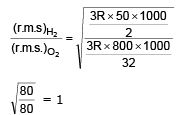QUESTION: 2

### X ml of H2 gas effuses through a hole in a container in 5 sec. The time taken for the effusion of the same volume of the gas specified below under identical conditions is :

Solution: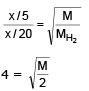M = 2 × 16
M = 32
i.e., = O2

QUESTION: 3

### One mole of N2O4 (g) at 300 k is kept in a closed container under one atmp. It is heated to 600 k when 20 % by mass of N2O4 (g) decomposes to NO2 (g) . The resultant pressure is :

Solution:

20% by mass decomposition
⇒ mole decomposed aslo 20%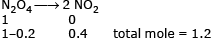Initially 1×V = 1×R×300
finally P×V = (1.2)×R×600
⇒ 2.4 atm

QUESTION: 4

According to Graham's law , at a given temperature the ratio of the rates of diffusion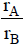of gases A and B is given by :

Solution: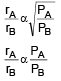QUESTION: 5

A gas will approach ideal behaviour at :

Solution:

A gas will be have ideally at low pressure & high temperature.

QUESTION: 6

The compressibility of a gas is less than unity at STP. Therefore

Solution: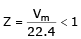⇒ Vm = 22.4

QUESTION: 7

The r. m. s. velocity of hydrogen is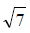times the r. m. s. velocity of nitrogen. If T is the temperature of the gas :

Solution: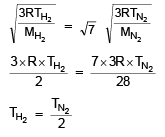QUESTION: 8

The root mean square velocity of an ideal gas at constant pressure varies with density as

Solution: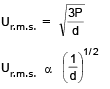*Multiple options can be correct
QUESTION: 9

Select the correct option(s) for an ideal gas

Solution: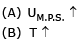*Multiple options can be correct
QUESTION: 10

Select the correct observation for a 8.21 lit container, filled with 2 moles of He at 300 K.,

Solution:

V = 8.21 L
n = 2
T = 300 K
(A) P = 6 atm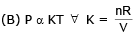*Multiple options can be correct
QUESTION: 11

For 2 mol of CO2 gas at 300 K

Solution:

Transtational K. E. =3/2 nRT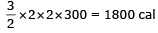*Multiple options can be correct
QUESTION: 12

Match the entries in column I with entries in Column II and then pick out correct options.

Column I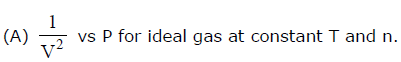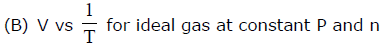(C) log P vs log V for ideal gas at constant T and n.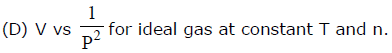Column II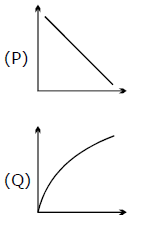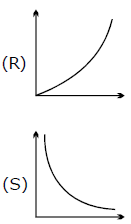Solution:
*Multiple options can be correct
QUESTION: 13

Select the incorrect statement(s):

Solution:

incorrect (A) at boyle’s temperture a real gas behave as ideal irresp. of pressur (B) At critical condn a real gas behave as ideal.

*Multiple options can be correct
QUESTION: 14

Match the description in Column I with graph provided in Column II. For n moles of ideal gas at emperature
'T'.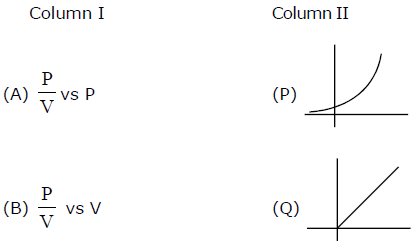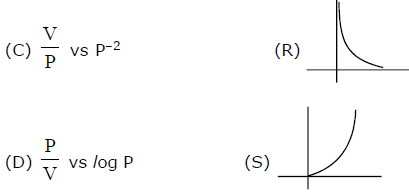Solution:

(A) PV = nRt
Pv = K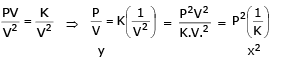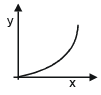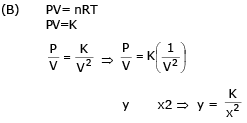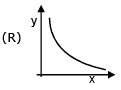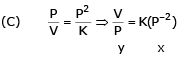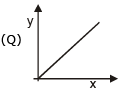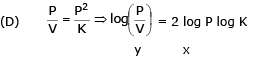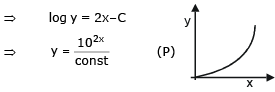*Multiple options can be correct
QUESTION: 15

Select the correct option for an ideal gas undergoing a process as shown in diagram.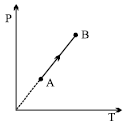Solution: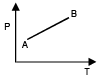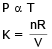n = chang ; V = must change to maintain
n = const; V constant

*Multiple options can be correct
QUESTION: 16

Two containers X & Y are present with container X consisting of some mass of He at some temperature while container Y having double the volume as that of container X & kept at same temperature containing same mass of H2 gas as the mass of Helium gas. Based on this data & the following conditions answer the question that follows

Assume sizes of H2 molecule & He atom to be same & size of H–atom to be half to that of He–atom & only bimolecular collisions to be occuring.

Condition I: all except one atom of He are stationary in cont. X & all molecules of H2 are moving in container Y.

Condition II: both containers contain all moving molecules

Q.

Assuming condition I to be applicable & if no. of total collisions occuring per unit time is 'A' in container X then no. of total collisions made by any one molecule in container Y will be:

Solution:
*Multiple options can be correct
QUESTION: 17

Two containers X & Y are present with container X consisting of some mass of He at some temperature while container Y having double the volume as that of container X & kept at same temperature containing same mass of H2 gas as the mass of Helium gas. Based on this data & the following conditions answer the question that follows

Assume sizes of H2 molecule & He atom to be same & size of H–atom to be half to that of He–atom & only bimolecular collisions to be occuring.

Condition I: all except one atom of He are stationary in cont. X & all molecules of H2 are moving in container Y.

Condition II: both containers contain all moving molecules

Q.

Assuming condition II then ratio of 'total no. of collisions per unit volume per unit time' in container X & container Y is (container X : container Y)

Solution:
*Multiple options can be correct
QUESTION: 18

Select the correct option(s):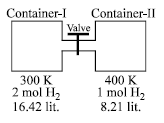Solution: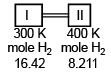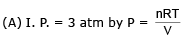(B) Pressure just after openin doesn’t changes
(D) Pressure becomes same after some time

*Multiple options can be correct
QUESTION: 19

Two containers X & Y are present with container X consisting of some mass of He at some temperature while container Y having double the volume as that of container X & kept at same temperature containing same mass of H2 gas as the mass of Helium gas. Based on this data & the following conditions answer the question that follows

Assume sizes of H2 molecule & He atom to be same & size of H–atom to be half to that of He–atom & only bimolecular collisions to be occuring.

Condition I: all except one atom of He are stationary in cont. X & all molecules of H2 are moving in container Y.

Condition II: both containers contain all moving molecules

Q.

Assuming condition II to be applicable, if temperature only of container Y is doubled to that of original (causing dissociation of all H2 gas into H gaseous atoms) then, if no. of total collisions per unit volume per unit time in container X is A then, no. of 'total collisions made by all molecules per unit volume in container Y would be

Solution:
*Multiple options can be correct
QUESTION: 20

On the recently discovered 10th planet it has been found that the gases follow the relationship PeV/2 = nCT where C is constant other notation are as usual (V in lit., P in atm and T in Kelvin). A curve is plotted between P and V at 500 K & 2 moles of gas as shown in figure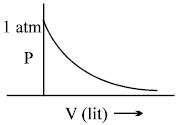The value of constant C is

Solution:

Pev/2 = nCT
T = 500 K
n = 2 moles
P = 1 atm
on solving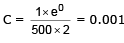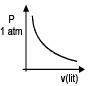*Multiple options can be correct
QUESTION: 21

An ideal gas having 2 moles (fixed) is subjected to the changes as shown is (P-V) diagram. Select the correct option(s) from the following diagram.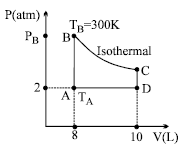Solution:
*Multiple options can be correct
QUESTION: 22

An open ended mercury manometer is used to measure the pressure exerted by a trapped gas as shown in the figure. Initially manometer shows no difference in mercury level in both columns as shown in diagram.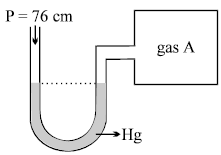After sparking 'A' dissociates according to following reaction

2A(g) → 3B(g) + 2C(g)

If pressure of Gas "A" decreases to 0.8 atm. Then (Assume temperature to be constant and is 300 K)

Solution:
*Multiple options can be correct
QUESTION: 23

On the recently discovered 10th planet it has been found that the gases follow the relationship PeV/2 = nCT where C is constant other notation are as usual (V in lit., P in atm and T in Kelvin). A curve is plotted between P and V at 500 K & 2 moles of gas as shown in figureQ.

Find the slope of the curve plotted between P Vs T for closed container of volume 2 lit. having same moles of gas

Solution: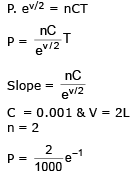*Multiple options can be correct
QUESTION: 24

On the recently discovered 10th planet it has been found that the gases follow the relationship PeV/2 = nCT where C is constant other notation are as usual (V in lit., P in atm and T in Kelvin). A curve is plotted between P and V at 500 K & 2 moles of gas as shown in figure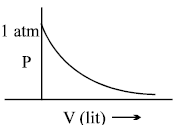Q.

If a closed container of volume 200 lit. of O2 gas (ideal gas) at 1 atm & 200 k is taken to planet. Find the pressure of oxygen gas at the planet at 821 k in same container

Solution: